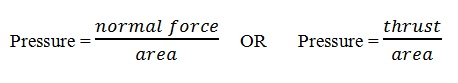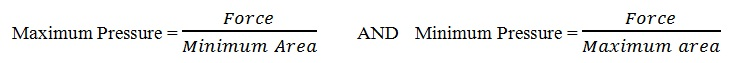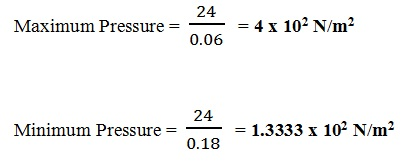# Pressure | Physics Notes

### Guest Account

Attempt Revision Exercises on Pressure
Attempt Form One Physics Questions
Guest Account

# Pressure

- defined as the force acting normally (perpendicularly) per unit area
- The SI units for pressure is newton per metre squared (N/m² or Nm-2)
. - One Nm-2 is known as one Pascal (Pa).

#### Formula for Pressure- pressure is also measured in another unit called bar. 1 bar = 105N/m², 1 millibar = 100N/m²

#### Maximum and Minimum Pressure##### Examples: Calculating Pressure
A rectangular brick of weight 24 N, measures 60 cm × 20 cm × 30 cm. calculate the values of the maximum and minimum pressures which the block exert when resting on a horizontal table.
Solution
Area of the smallest face = 0.2 × 0.3 = 0.06 m²
Area of the largest face = 0.6 × 0.3 = 0.18 m²### Types of forces

1. Gravitational force –this is the force of attraction between two bodies of given masses.
- Earth's gravitational force is the force which pulls a body towards its center. This pull of gravity is called weight.
2. Force of friction – this is a force which opposes the relative motion of two surfaces in contact with each other. Friction in fluids is known as viscosity.
3. Tension force – this is the pull or compression of a string or spring at both its ends.
4. Upthrust force – this is the upward force acting on an object immersed in a fluid.
5. Cohesive and adhesive forces – cohesive is the force of attraction of molecules of the same kind while adhesive is the force of attraction of molecules of different kinds.
6. Magnetic force – this is a force which causes attraction or repulsion in a magnet.
7. Electrostatic force – this is the force of attraction or repulsion of static charges.
8. Centripetal force – this is a force which constrains a body to move in a circular orbit or path.
9. Surface tension – this is the force which causes the surface of a liquid to behave like a stretched skin. This force is cohesive.

Factors affecting surface tension
a) Impurities – they reduce the surface tension of a liquid i.e. addition of detergent.
b) Temperature – rise in temperature reduces tension by weakening inter-molecular forces.

### Mass and weight

Mass is the amount of matter contained in a substance while weight is the pull of gravity on an object.
The SI unit for mass is the Kg while weight is the newton (N). Mass is constant regardless of place while weight changes with place.
Weight = Mass x g, where g is the gravitational force

#### Differences between mass and weight Mass

Mass
It is the quantity of matter in a body
It is measured in kilograms
It is the same everywhere
It is measured using a beam balance
Has magnitude only Weight

Weight
It is the pull of gravity on a body
It is measured in newton's
It changes from place to place
Measured using a spring balance
Has both magnitude and direction

-- select another example --

#### Measuring Force

A spring balance is the most common tool for measuring force.
The length of a spring is 16.0 cm. its length becomes 20.0 cm when supporting a weight of 5.0 N. Calculate the length of the spring when supporting a weight of 6.0 N.

Solution
5N stretches to a length of (20.0 - 16.0) = 4cm
hence 1N = 4 ÷ 5 = 0.8 cm
Therefore, 6.0 x 0.8 = 4.8cm
Overall length will be: 16.0 + 4.8 = 20.8cm
= 20.8

### Vector and Scalar Quantities

A scalar quantity is a quantity which has magnitude (size) only . Examples are distance, mass, speed

A vector quantity is a quantity which has both magnitude and direction. Examples are displacement, weight, velocity